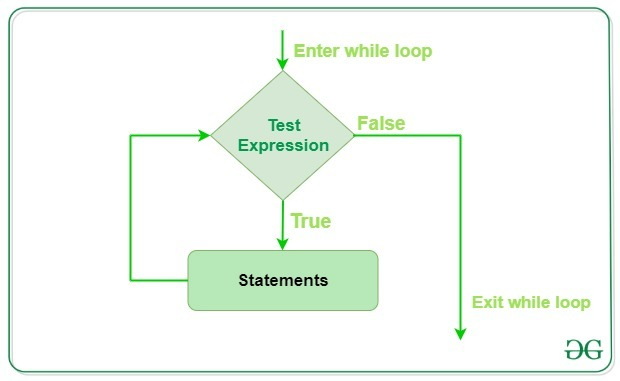# Python While Loops

In Python, While Loops is used to execute a block of statements repeatedly until a given condition is satisfied. And when the condition becomes false, the line immediately after the loop in the program is executed. While loop falls under the category of indefinite iteration. Indefinite iteration means that the number of times the loop is executed isn’t specified explicitly in advance.

Syntax:

```while expression:
statement(s)
```

Statements represent all the statements indented by the same number of character spaces after a programming construct are considered to be part of a single block of code. Python uses indentation as its method of grouping statements. When a while loop is executed, `expr `is first evaluated in a `Boolean context` and if it is true, the loop body is executed. Then the `expr `is checked again, if it is still true then the body is executed again and this continues until the expression becomes false.

Example:

 `# Python program to illustrate  ` `# while loop  ` `count ``=` `0` `while` `(count < ``3``):      ` `    ``count ``=` `count ``+` `1` `    ``print``(``"Hello Geek"``)  ` ` `  `print``() ` ` `  `# checks if list still ` `# contains any element  ` `a ``=` `[``1``, ``2``, ``3``, ``4``] ` `while` `a: ` `    ``print``(a.pop()) `

Output:

```Hello Geek
Hello Geek
Hello Geek

4
3
2
1
```

Working of While Loop :## Single statement while block

Just like the `if` block, if the `while` block consists of a single statement the we can declare the entire loop in a single line. If there are multiple statements in the block that makes up the loop body, they can be separated by semicolons `(;)`.

 `# Python program to illustrate  ` `# Single statement while block  ` `count ``=` `0` `while` `(count < ``5``): count ``+``=` `1``; ``print``(``"Hello Geek"``) `

Output:

```Hello Geek
Hello Geek
Hello Geek
Hello Geek
Hello Geek
```

## Loop Control Statements

Loop control statements change execution from its normal sequence. When execution leaves a scope, all automatic objects that were created in that scope are destroyed. Python supports the following control statements.

• Continue Statement: It returns the control to the beginning of the loop.

 `# Prints all letters except 'e' and 's'  ` `i ``=` `0` `a ``=` `'geeksforgeeks'` ` `  `while` `i < ``len``(a):  ` `    ``if` `a[i] ``=``=` `'e'` `or` `a[i] ``=``=` `'s'``:  ` `        ``i ``+``=` `1` `        ``continue` `    ``print``(``'Current Letter :'``, a[i]) ` `    ``i ``+``=` `1`

Output:

```Current Letter : g
Current Letter : k
Current Letter : f
Current Letter : o
Current Letter : r
Current Letter : g
Current Letter : k
```
• Break Statement: It brings control out of the loop.

 `# break the loop as soon it sees 'e'   ` `# or 's' ` `i ``=` `0` `a ``=` `'geeksforgeeks'` ` `  `while` `i < ``len``(a):  ` `    ``if` `a[i] ``=``=` `'e'` `or` `a[i] ``=``=` `'s'``:  ` `        ``i ``+``=` `1` `        ``break` `    ``print``(``'Current Letter :'``, a[i]) ` `    ``i ``+``=` `1`

Output:

```Current Letter : g
```
• Pass Statement: We use pass statement to write empty loops. Pass is also used for empty control statements, functions and classes.

 `# An empty loop  ` `a ``=` `'geeksforgeeks'` `i ``=` `0` ` `  `while` `i < ``len``(a): ` `    ``i ``+``=` `1` `    ``pass` `print``(``'Value of i :'``, i) `

Output:

```Value of i : 13
```

## while-else loop

As discussed above, while loop executes the block until a condition is satisfied. When the condition becomes false, the statement immediately after the loop is executed.
The else clause is only executed when your while condition becomes false. If you break out of the loop, or if an exception is raised, it won’t be executed.

Note: The else block just after for/while is executed only when the loop is NOT terminated by a break statement.

 `# Python program to demonstrate ` `# while-else loop ` `i ``=` `0`  `while` `i < ``4``: ` `    ``i ``+``=` `1` `    ``print``(i)  ` `else``: ``# Executed because no break in for  ` `    ``print``(``"No Break\n"``)  ` ` `  `i ``=` `0`  `while` `i < ``4``:  ` `    ``i ``+``=` `1` `    ``print``(i)  ` `    ``break` `else``: ``# Not executed as there is a break  ` `    ``print``(``"No Break"``) `

Output:

```1
2
3
4
No Break

1
```

My Personal Notes arrow_drop_upCheck out this Author's contributed articles.

If you like GeeksforGeeks and would like to contribute, you can also write an article using contribute.geeksforgeeks.org or mail your article to contribute@geeksforgeeks.org. See your article appearing on the GeeksforGeeks main page and help other Geeks.

Please Improve this article if you find anything incorrect by clicking on the "Improve Article" button below.

Article Tags :

2

Please write to us at contribute@geeksforgeeks.org to report any issue with the above content.